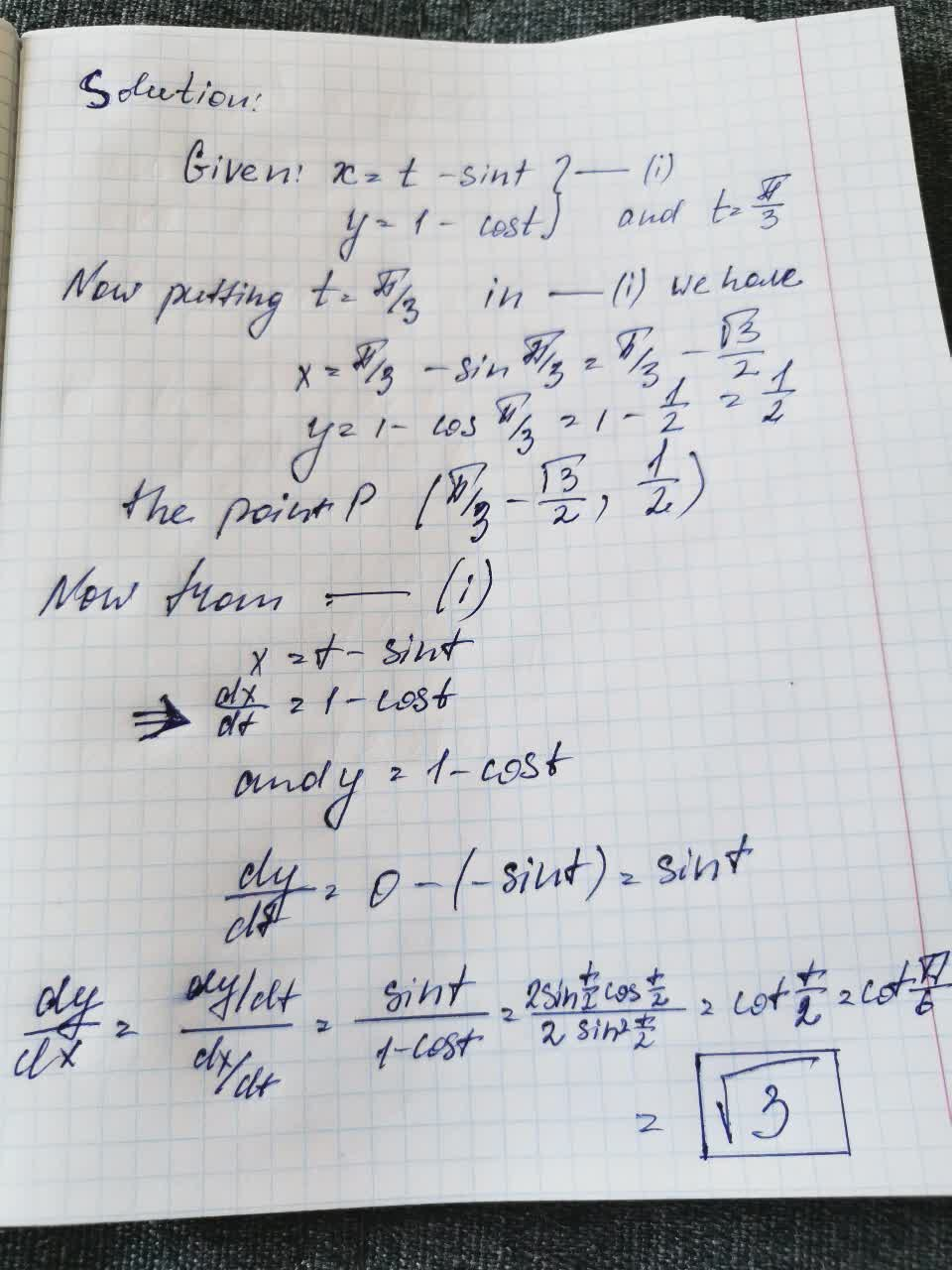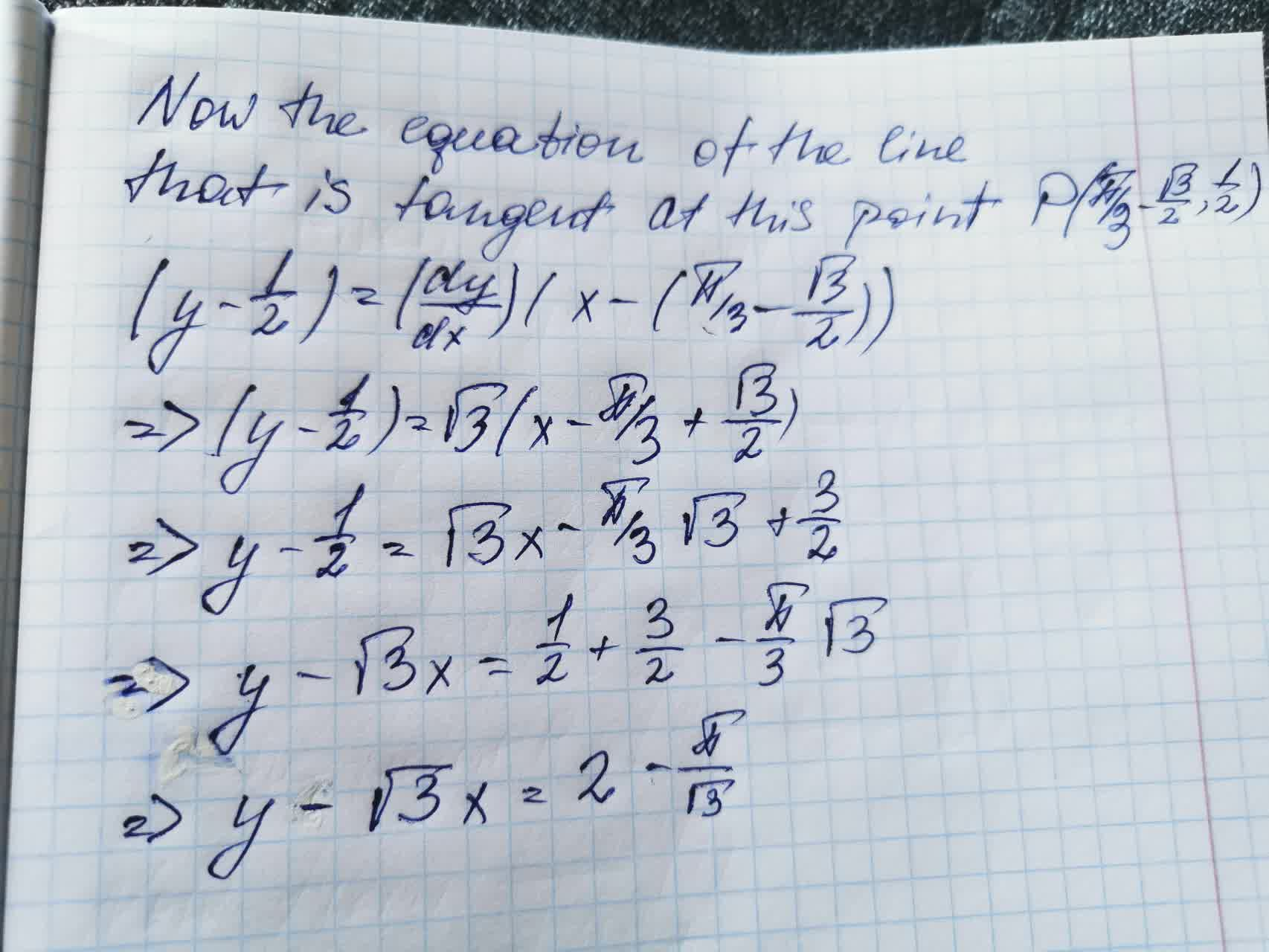# x=t-sinty=1-cost to the curve given by the parametric equation t=\frac{\pi}{3Reeves 2021-08-29 Answered

$$x=t-\sin t$$
$$y=1-\cos t$$ to the curve given by the parametric equation
$$\displaystyle{t}={\frac{{\pi}}{{{3}}}}$$ find teh equation of the line that is tangent at its point

• Questions are typically answered in as fast as 30 minutes

### Plainmath recommends

• Get a detailed answer even on the hardest topics.
• Ask an expert for a step-by-step guidance to learn to do it yourself.krolaniaN

Given that: